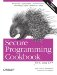# Performing Set Operations on Sequences

Problem

You have sequences that you want to rearrange using set operations like union, difference, or intersection.

Solution

Use the standard library functions built for exactly this purpose: set_union , set_dif-ference , and set_intersection . Each of these performs its respective set operation and places the results in an output range. See how to do this in Example 7-8.

Example 7-8. Using set operations

```#include
#include
#include
#include
#include
#include "utils.h" // For printContainer( ): see 7.10

using namespace std;

int main( ) {

cout << "Enter some strings: ";
istream_iterator start(cin);
istream_iterator end;
set s1(start, end);

cin.clear( );

cout << "Enter some more strings: ";
set s2(++start, end);

set setUnion;
set setInter;
set setDiff;

set_union(s1.begin( ), s1.end( ),
s2.begin( ), s2.end( ),
inserter(setUnion, setUnion.begin( )));

set_difference(s1.begin( ), s1.end( ),
s2.begin( ), s2.end( ),
inserter(setDiff, setDiff.begin( )));

set_intersection(s1.begin( ), s1.end( ),
s2.begin( ), s2.end( ),
inserter(setInter, setInter.begin( )));

cout << "Union:
";
printContainer(setUnion);
cout << "Difference:
";
printContainer(setDiff);
cout << "Intersection:
";
printContainer(setInter);
}```

The output to this program looks like this (printContainer just prints the contents of a container):

```Enter some strings: a b c d
^Z
Enter some more strings: d e f g
^Z
Union: a b c d e f g
Difference: a b c
Intersection: d```

Discussion

The set operations in the standard library all look and work pretty much the same. Each takes two ranges, performs its respective operation on them, and places the results in an output iterator. You have to make sure there is enough room in the output sequence, or use an inserter or a back_inserter (see the discussion in Recipe 7.5 to see how to use a back_inserter).

The declaration for set_union looks like this:

`Out set_union(In first1, In last1, In first2, In last2, Out result)`

The declarations for set_difference, set_intersection, and set_symmetric_difference all look the same.

To use these functions, do as I did in Example 7-8. To find the intersection of two sets, for example, you might call set_intersection like this:

```set_intersection(s1.begin( ), s1.end( ),
s2.begin( ), s2.end( ),
inserter(setInter, setInter.begin( )));```

The last argument to set_intersection needs further explanation. inserter is a function template defined in that takes a container and an iterator and returns an output iterator that calls insert on its first argument when values are assigned to it. If you use it on a sequence container, it inserts values before the iterator you pass in as its last argument. If you use it on an associative container as I did in the previous code snippet, this iterator is ignored and elements are inserted where they belong according to the container's sort criteria.

sets are a convenient example for my purposes, but you can call the set operations on any sequence, not just sets. For example, you may have lists that you want to do some set operations on:

```list lst1, lst2, lst3;

// Fill them with data

lst1.sort( );// Elements must be sorted
lst2.sort( );

set_symmetric_difference(lst1.begin( ), lst1.end( ),
lst2.begin( ), lst2.end( ),
back_inserter(lst3));```

However, since lists are not stored in sorted order, you have to sort them first or the results of the set operations are invalid. Notice also that I used a back_inserter in this example instead of an inserter. back_inserter works similarly to inserter, except that it uses push_back to add elements to the container you give it. You don't need to do it this way; for example, you could resize the output container so that it's always big enough:

```lst3.resize(lst1.size( ) + lst2.size( ));

set_symmetric_difference(lst1.begin( ), lst1.end( ),
lst2.begin( ), lst2.end( ),
lst3.begin( ));```

If the output sequence is large enough, you can just pass in an iterator pointing to the first element in the sequence with begin.

In case you don't know what set_symmetric_difference is, I'll tell you. It's the union of the differences of two sets in opposite order. That is, if a and b are sets, the symmetric difference is a - bb - a. Another way to put it is to say that the symmetric difference is the set of all elements that appear in one set but not the other.

There's one more thing you should know about the set operations. Since sequences don't have to be unique, you can have a "set" with duplicate values. Strictly speaking, of course, mathematical sets can't contain duplicates, so this may not be intuitive. Consider what the output of Example 7-8 might look like if I used lists instead of sets (you can enter duplicate values when running Example 7-8, but they aren't added to the sets because set::insert fails when the element being inserted already exists in the set):

```Enter some strings: a a a b c c
^Z
Enter some more strings: a a b b c
^Z
Union: a a a b b c c
Difference: a c
Intersection: a a b c```

What's happening here is that the set operations iterate through both sequences and compare corresponding values to determine what to put in the output sequence.

Finally, the set operations in their default form (using operator< to compare elements) probably don't work like you want them to if your sets contain pointers. To get around this, write a functor that compares pointers' objects, as in Recipe 7.4.

Recipe Recipe 7.4Secure Programming Cookbook for C and C++: Recipes for Cryptography, Authentication, Input Validation & More
ISBN: 0596003943
EAN: 2147483647
Year: 2006
Pages: 241